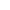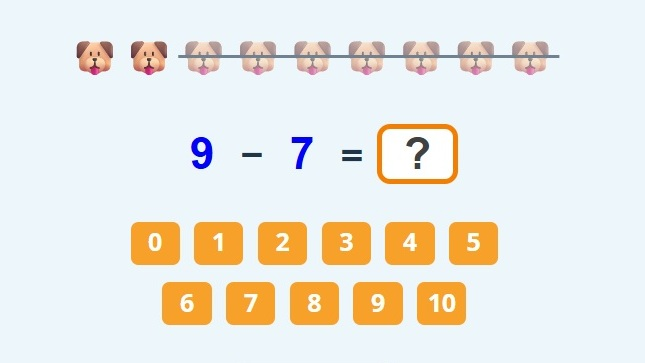﻿ Single digit subtraction within 10: worksheets and games

# Single digit subtraction within 10: worksheets and games

Play subtraction within 10 games online. Download free printable subtraction with pictures worksheet in PDF. simple subtraction worksheets with pictures. subtraction within 10 worksheet PDF.

New gameFill in the result of single digit subtraction to the empty box.

## Subtraction with pictures for KindergartenCute pictures help kids to solve single digit subtraction more easily. The total amount of pictures represents the largest number. From this number kids remove crossed out pictures. What remains is the answer.

Insert the result of the single digit subtraction to the empty box. Press "Check it" to find out if your answer is correct. If your result of subtraction with pictures is OK, the box will be green. If your answer isn´t correct, the box will be red and you will see the correct number.

Press the button "New game" and play another single digit subtraction game.

Subtraction with pictures game teaches kids in Kindergarten to subtract numbers up to 10. You can play subtraction within 10 games online.

Do you want to try something more difficult? Then play subtraction within 20 game or this subtraction pyramid game.

Watch this nice tutorial on YouTube which teaches kids basics of subtraction.

## Single digit subtraction worksheets with pictures in PDF

Click the picture below and download our free printable single digit subtraction worksheets with pictures in PDF. You can find more kindergarten worksheets here.